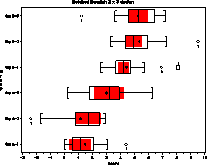boxplot boxplot - SAS/Graph Box and Whisker plot boxplot

# SAS Macro Programs: boxplot

\$Version: 2.1 (09 Jan 2005)
Michael Friendly
York University

## BOXPLOT macro (get boxplot.sas)

The BOXPLOT macro draws side-by-side boxplots for the groups defined by one or more grouping (CLASS) variables in a data set. The boxplots may be formatted horizontally or vertically, they may be shown with "notches", indicating approximate 95% confidence intervals for difference in medians, and the groups may be ordered in a variety of ways.

### Parameters

DATA=_LAST_
Name of the input data set.
CLASS=
Grouping variable(s). The CLASS= variables may be character or numeric. If the CLASS= variable is a character variable, or there is more than one CLASS= variable, the macro automatically constructs a SAS format to label the group.
VAR=
The name of the response variable to be plotted. The VAR= variable is plotted on the ordinate when ORIENT=V and on the abscissa when ORIENT=H.
ID=
A character variable to identify each observation. If an ID= variable is specified, outside variables are labelled on the graph, using the first 8 characters of the value of the ID variable (to reduce overplotting). Otherwise, outside points are not labelled.
SORTBY=
Specifies a variable or statistic keyword used to order the levels of the CLASS= variables along the class axis. If SORTBY= is not specified, the classes are ordered along the axis in sorted order (using the format specified by CLASSFMT= if that has been used). Otherwise, you can specify the name of a variable in the dataset, or one of the statistics, _MEAN_, _MEDIAN_, N (no underscores), _Q1_, or _Q3_ and the classes will be ordered on the axis according to the values of that statistic for each group.
width=.5
Box width as proportion of the maximum. The default, width=.5, means that the maximum box width is half the spacing between boxes.
NOTCH=0
Specifies whether or not to draw notched boxes. 1=draw notched boxes; 0=do not.
CBOX=BLACK
CFILL=
Color for box fill. If specified, the area inside the box outline is filled using a solid pattern and the CFILL color. A light grey, e.g., CFILL=GRAYD0 is often pleasing.
CNOTCH=
Color for notch fill. If specified, the area between the upper/lower notches is filled using a solid pattern and the CNOTCH color. This is often an alternative to drawing explicit notches.
LBOX=1
Line style for box outline
ORIENT=V
Box orientation: V gives vertical boxes, H gives horizontal boxes. If the labels for the CLASS= variables are longish, horizontal boxes are preferred, since the labels will appear on the Y axis.
CONNECT=0
Specifies the line style used to connect medians of adjacent groups. If CONNECT=0, the medians of adjacent groups are not to be connected.
F=0.5
For a notched boxplot, the parameter F determines the notch depth, from the center of the box as a fraction of the halfwidth of each box. F must be between 0 and 1; the larger the value, the less deep is the notch.
FN=1
Box width proportionality factor. The default, FN=1 means all boxes are the same width. If you specify FN=sqrt(n), the boxes width will be proportional to the square root of the sample size of each group. Other functions of n are possible as well.
VARFMT=
The name of a format for the VAR= analysis variable.
CLASSFMT=
The name of a format for the class variable(s). If the CLASS variable is a character variable, or there are two or more CLASS variables, the program maps the sorted values of the class variable(s) into the integers 1, 2, ... levels, where levels is the number of distinct values of the class variable(s). A format provided for CLASSFMT should therefore provide labels corresponding to the numbers 1, 2, ... levels.
VARLAB=
Label for the analysis variable. If not specifed, the analysis axis is labelled with the variable name.
CLASSLAB=
Label for the class variable(s) used to label the axis.
XORDER=
Tick marks, and range for the horizontal axis, in the form XORDER = low TO high BY tick. With ORIENT=V, the horizontal axis pertains to the CLASS= variable(s); with ORIENT=H, the horizontal axis pertains to the VAR= analysis variable.

When there are very few (3 or less) levels of the CLASS= variable, SAS/GRAPH often has problems dealing with the annotation information and labels. Setting XORDER= (or YORDER=, with ORIENT=H) to include an extra value at both ends of the range usually helps cure this problem. For example, if there are only two groups, with class values 1 and 2, set XORDER=0 to 3 by 1.

YORDER=
Tick marks, and range for ordinate, in the form YORDER = low TO high BY tick.
ANNO=
The name of an (optional) additional ANNOTATE data set to be used in drawing the plot. This requires some knowledge of the contents of the ANNOTATE data set, which you can see by specifying PRINT=ANNO in a previous call.
PRINT=OUTSIDE
Printed output: any one or more of ANNO, OUTSIDE, STATS.
OUT=BOXSTAT
Name of the output data set containing statistics used in drawing the boxplot. There is one observation for each group. The variables are N, _MEAN_, _MEDIAN_, _Q1_, _Q3_, _IQR_, LO_NOTCH, HI_NOTCH, LO_WHISK, HI_WHISK.
NAME=BOXPLOT
The name assigned to the graph in the graphic catalog.

### GOPTIONS

If there are many groups and/or the formatted labels of group names are long, you may need to increase the HPOS= option to allow a sufficient number of character positions for the labels.

The font face and size used for labels is controlled by the global graphics options FTEXT and HTEXT.

### Example

The example below plots data for 6 groups in a 2 x 3 design, classified by factors A and B. No explicit notches are drawn, but the region between the notches is filled in red.
```title h=1.5 'Notched Boxplot: 2 x 3 design';
%boxplot(data=testbox,
class= A B,
var=y,
id=name,
notch=0,                    /* 1=do notched boxplots        */
classfmt=ab.,               /* Format for class variables   */
varlab=Score,
classlab=Group,
lbox=33,
cnotch=red,
orient=H);
```